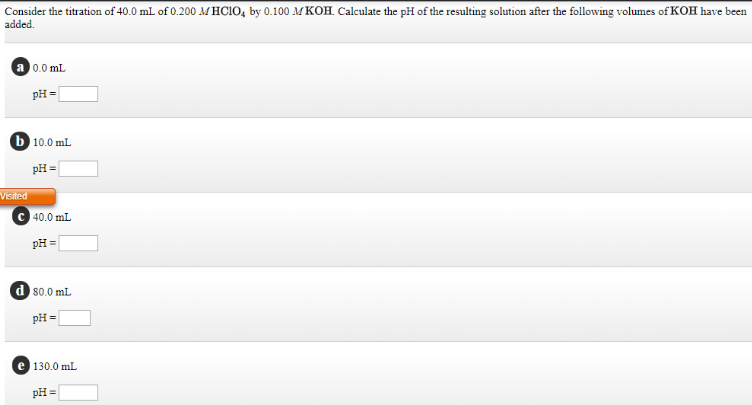# Consider the titration of 40.0 mL of 0.200 M HClO4 by 0.100 M KOH. Calculate the pH of the resulting solution after the following volumes of KOH have been added. a. 0.0 mL pH = ___ b. 10.0 mL pH = ___ c. 40.0 mL pH = ___ d. 80.0 mL pH = ___ e. 130.0 mL pH = ___